# Creating and analyzing a SCIA model

caution

For the section "Performing an analysis" a SCIA worker is required.

This tutorial explains how a SCIA model can be created within VIKTOR, which can be used for analysis using an external coupling. The SCIA model that we will be constructing consists of a foundation slab with piles.

• The starting app for this tutorial can be downloaded here.
• The end result can be downloaded here.

Download the starting app to your hard disk. Run the `install` command from within the app folder to install the app and its dependencies, `clear` any previous saved local database, and run `start` to start to app:

``viktor-cli installviktor-cli clearviktor-cli start``

In the browser, click the "Example" entity to open its editor and verify the app is installed and running as expected.

## Folder structure​

The app has the following folder structure:

``scia-tutorial├── app.py├── model.esa├── requirements.txt└── viktor.config.toml``
caution

The example template SCIA file (model.esa) in this tutorial has been made in SCIA 19.0. The file cannot be opened nor run with versions < 19.0 (and is not guaranteed to work for > 19.0).

If you are using a version of SCIA other than 19.0, a template file must be created for (and the worker must run) this specific version. This can be done with the following procedure:

1. Open a new project in your SCIA version of choice
2. Make sure that the material that will be used is present in the materials library
3. Setup the XML I/O document to export the desired results (see the SCIA documentation)
4. Save the empty file as model.esa
5. Replace the existing esa file with the just created one in the foundation folder

Open `app.py` and have a look at the code that we have set up for you. Note that the code already creates an empty SCIA model and some basic class structure. We will extend the code and add specific SCIA objects, step-by-step, throughout this tutorial.

## Creating the corner nodes​

If we assume that one of the corner points is located in the origin, the other three corner point locations can easily be calculated using `width_x` and `width_y`. Open `app.py` and add the following code `create_scia_model`:

``def create_scia_model(self, params) -> SciaModel:    model = SciaModel()    '''    STRUCTURE    '''    # create nodes at the slab corners    width_x = params.geometry.slab.width_x * 1e-03    width_y = params.geometry.slab.width_y * 1e-03    n1 = model.create_node('n1', 0, 0, 0)  # origin    n2 = model.create_node('n2', 0, width_y, 0)    n3 = model.create_node('n3', width_x, width_y, 0)    n4 = model.create_node('n4', width_x, 0, 0)    return model``
note

The corner nodes are defined in clockwise direction, since this order will be used later to create a 3D extrusion object.

While setting up a SCIA model in VIKTOR, the 3D visualization is a very helpful feature to verify that the written logic does indeed create the model as desired. Therefore, we will extend `create_visualization_geometries` to get and show the just created nodes. This can easily be done by calling the `nodes` attribute of the `SciaModel`:

``...from viktor import Colorfrom viktor.geometry import Point, Sphere, Materialdef create_visualization_geometries(self, params, scia_model):    geometries = []    for node in scia_model.nodes:        node_obj = Sphere(Point(node.x, node.y, node.z),  params.geometry.slab.width_y * 1e-05)        node_obj.material = Material('node', color=Color(0, 255, 0))        geometries.append(node_obj)    return geometries``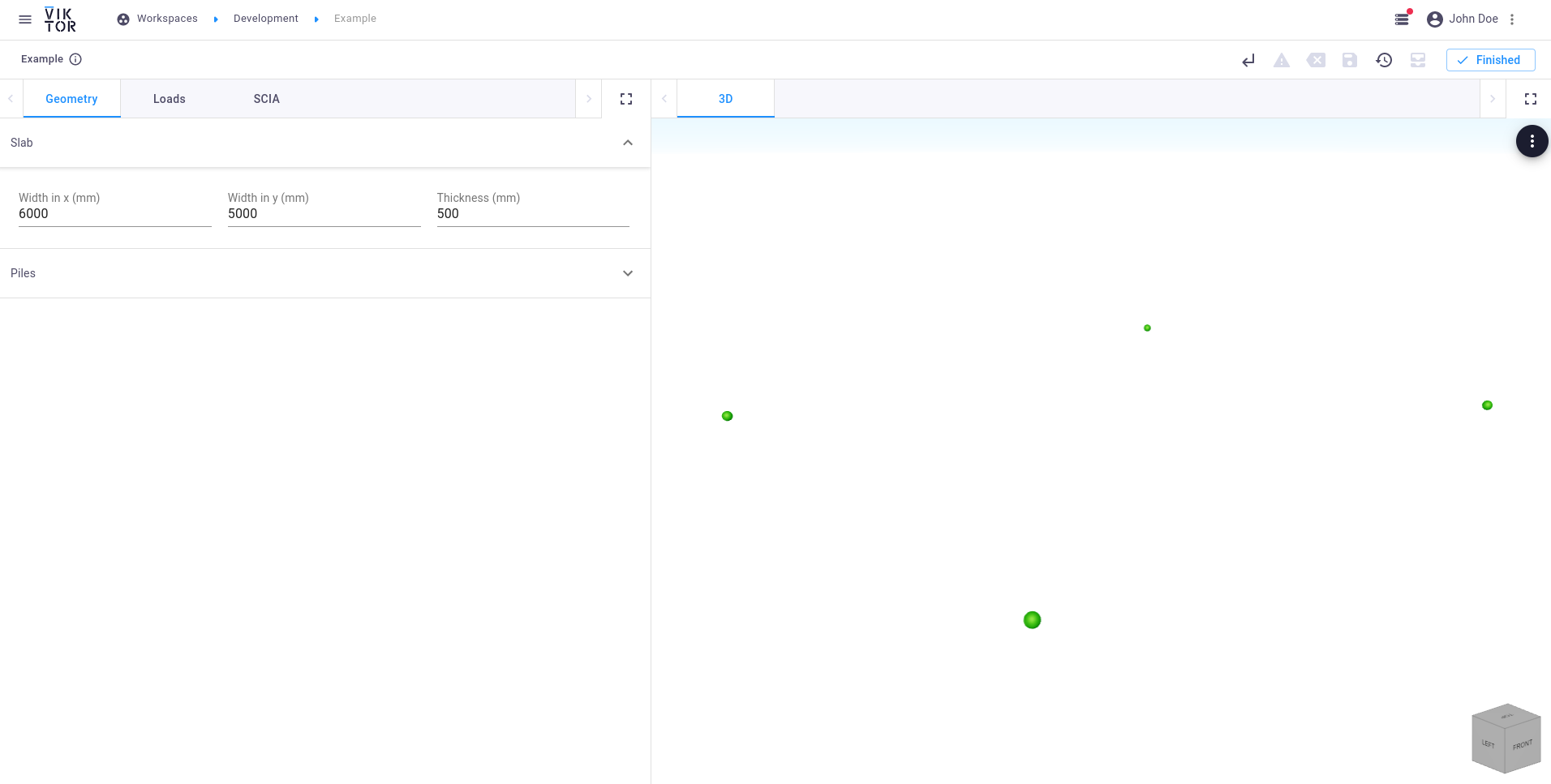Corner nodes

## Creating the piles​

For the purpose of this tutorial, some parameters like the number of piles and the distance between the piles and the edge are fixed. The locations of these piles however, depend on the dimensions of the slab:

``...import numpy as npimport itertoolsdef create_scia_model(self, params) -> SciaModel:    ...    # create the pile nodes    number_of_piles_x = 4    number_of_piles_y = 3    pile_edge_distance = 0.3    pile_length = params.geometry.piles.length    start_x = pile_edge_distance    end_x = width_x - pile_edge_distance    x = np.linspace(start_x, end_x, number_of_piles_x)    start_y = pile_edge_distance    end_y = width_y - pile_edge_distance    y = np.linspace(start_y, end_y, number_of_piles_y)    pile_positions = np.array(list(itertools.product(x, y)))    pile_top_nodes = []    pile_bottom_nodes = []    for pile_id, (pile_x, pile_y) in enumerate(pile_positions, 1):        n_top = model.create_node(f'K:p{pile_id}_t', pile_x, pile_y, 0)        n_bottom = model.create_node(f'K:p{pile_id}_b', pile_x, pile_y, -pile_length)        pile_top_nodes.append(n_top)        pile_bottom_nodes.append(n_bottom)    return model``

Since we already loop through all the nodes in the `create_visualization_geometries` method (`for node in scia_model.nodes:`), the nodes located at the pile ends will automatically be visualized when the app is (automatically) restarted.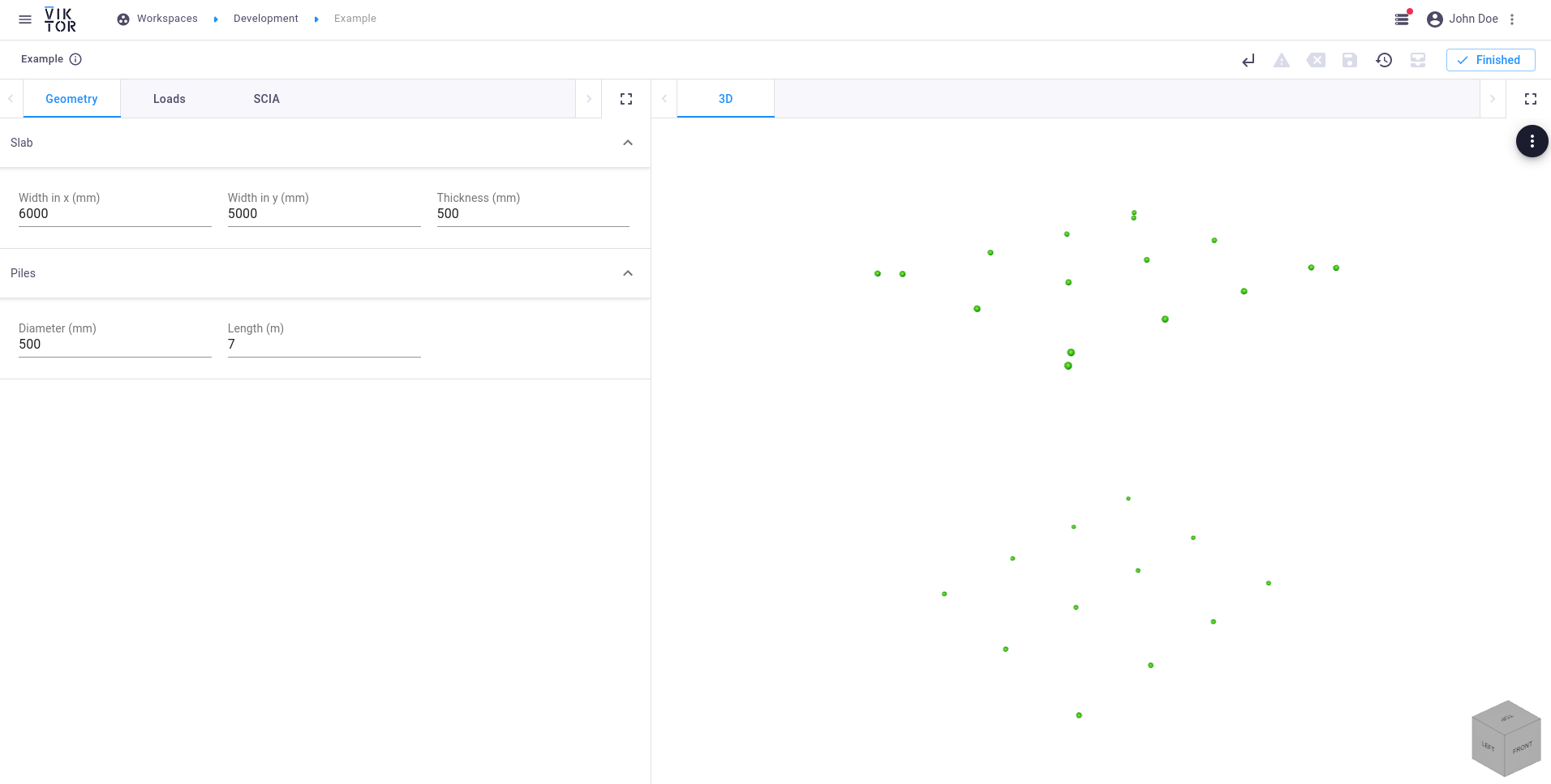Pile nodes

The top and bottom nodes of the piles can now be used along with the pile diameter defined in the `Parametrization` to create a circular cross-section and beam elements. The cross-section in turn needs a `SciaMaterial` as input, which needs to be defined in the .esa model that is used.

note

The `Material` from the SCIA package is imported with a different name to prevent conflicts with the `Material` import from `viktor.geometry`.

``...from viktor.external.scia import Material as SciaMaterialdef create_scia_model(self, params) -> SciaModel:    ...    # create pile beams    pile_diameter = params.geometry.piles.diameter * 1e-03    material = SciaMaterial(0, 'C30/37')    cross_section = model.create_circular_cross_section('concrete_pile', material, pile_diameter)    pile_beams = []    for pile_id, (n_top, n_bottom) in enumerate(zip(pile_top_nodes, pile_bottom_nodes), 1):        pile_beam = model.create_beam(n_top, n_bottom, cross_section)        pile_beams.append(pile_beam)    return model``

For the visualization of the piles, a `CircularExtrusion` is used. This object needs a diameter and `Line` object which specifies the direction of the beam in terms of a start and end `Point`.

``...from viktor.geometry import CircularExtrusion, Linedef create_visualization_geometries(self, params, scia_model):    ...   # pile beams    pile_diameter = params.geometry.piles.diameter * 1e-03    for beam in scia_model.beams:        point_top = Point(beam.begin_node.x, beam.begin_node.y, beam.begin_node.z)        point_bottom = Point(beam.end_node.x, beam.end_node.y, beam.end_node.z)        beam_obj = CircularExtrusion(pile_diameter, Line(point_top, point_bottom))        beam_obj.material = Material('beam', threejs_roughness=1, threejs_opacity=0.3)        geometries.append(beam_obj)    ...``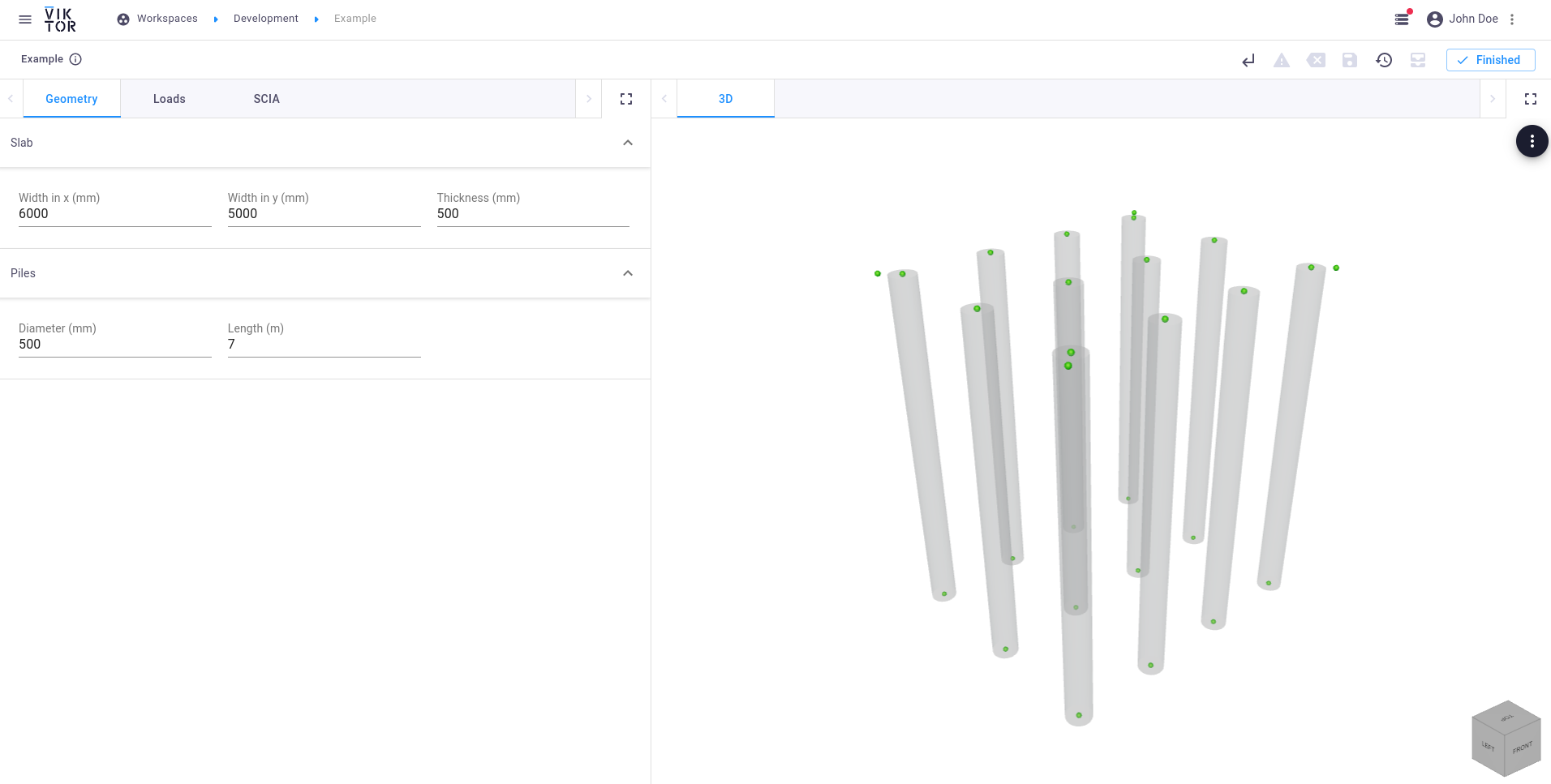Pile beams

## Creating the slab​

The corner nodes that were created earlier can be used to create the concrete slab object. Extend the `create_scia_model` method with the following lines:

``...from viktor.external.scia import Planedef create_scia_model(self, params) -> SciaModel:    ...    # create the concrete slab    material = SciaMaterial(0, 'concrete_slab')    thickness = params.geometry.slab.thickness * 1e-03    corner_nodes = [n1, n2, n3, n4]    slab = model.create_plane(corner_nodes, thickness, name='foundation slab', material=material)    return model``

Again we can verify the position and dimensions of the created slab by means of visualization:

``...from viktor.geometry import Extrusiondef create_visualization_geometries(self, params, scia_model):    ...    corner_points = [        Point(scia_model.nodes.x, scia_model.nodes.y, scia_model.nodes.z),        Point(scia_model.nodes.x, scia_model.nodes.y, scia_model.nodes.z),        Point(scia_model.nodes.x, scia_model.nodes.y, scia_model.nodes.z),        Point(scia_model.nodes.x, scia_model.nodes.y, scia_model.nodes.z),        Point(scia_model.nodes.x, scia_model.nodes.y, scia_model.nodes.z)    ]    thickness = params.geometry.slab.thickness * 1e-03    slab_obj = Extrusion(corner_points, Line(Point(0, 0, -thickness/2), Point(0, 0, thickness/2)))    slab_obj.material = Material('slab', threejs_roughness=1, threejs_opacity=0.3)    geometries.append(slab_obj)    ...``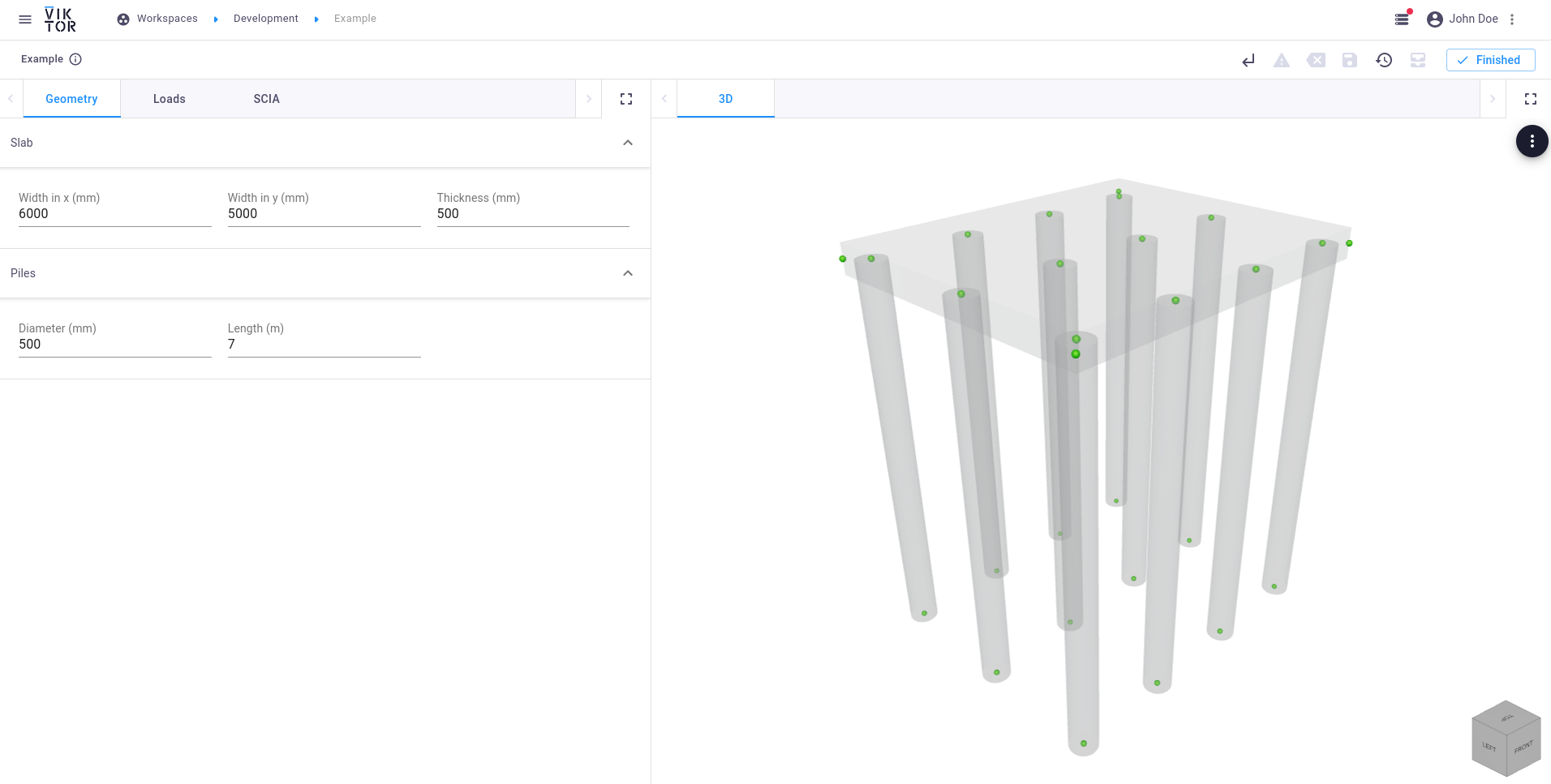Slab

## Creating the supports​

Since the foundation will be completely underground, the following supports have to be attached:

1. Point supports (`PointSupport`) at the bottom of each pile:

``...from viktor.external.scia import PointSupportdef create_scia_model(self, params) -> SciaModel:    ...    '''    SUPPORTS    '''    # create pile point supports    freedom_v = (        PointSupport.Freedom.FREE, PointSupport.Freedom.FREE, PointSupport.Freedom.FLEXIBLE,        PointSupport.Freedom.FREE, PointSupport.Freedom.FREE, PointSupport.Freedom.FREE    )    kv = 400 * 1e06    stiffness_v = (0, 0, kv, 0, 0, 0)    for pile_id, pile_beam in enumerate(pile_beams, 1):        n_bottom = pile_beam.end_node        model.create_point_support(f'Sn:p{pile_id}', n_bottom, PointSupport.Type.STANDARD,                                   freedom_v, stiffness_v, PointSupport.CSys.GLOBAL)    return model``
2. Line supports (`LineSupport`) on the piles:

``...from viktor.external.scia import LineSupportdef create_scia_model(self, params) -> SciaModel:    ...    # create pile line supports    kh = 10 * 1e06    for pile_id, pile_beam in enumerate(pile_beams, 1):        model.create_line_support_on_beam(pile_beam, x=LineSupport.Freedom.FLEXIBLE, stiffness_x = kh,                                          y=LineSupport.Freedom.FLEXIBLE, stiffness_y = kh,                                          z=LineSupport.Freedom.FREE, rx=LineSupport.Freedom.FREE,                                          ry=LineSupport.Freedom.FREE, rz=LineSupport.Freedom.FREE,                                          c_sys=LineSupport.CSys.GLOBAL)    return model``
3. Line supports (`LineSupport`) on the slab edges:

``...def create_scia_model(self, params) -> SciaModel:    ...    # create the slab supports    stiffness_x = 50 * 1e06    stiffness_y = 50 * 1e06    for edge in (1, 3):        model.create_line_support_on_plane((slab, edge),                                           x=LineSupport.Freedom.FLEXIBLE, stiffness_x=stiffness_x,                                           y=LineSupport.Freedom.FREE,                                           z=LineSupport.Freedom.FREE,                                           rx=LineSupport.Freedom.FREE,                                           ry=LineSupport.Freedom.FREE,                                           rz=LineSupport.Freedom.FREE)    for edge in (2, 4):        model.create_line_support_on_plane((slab, edge),                                           x=LineSupport.Freedom.FREE,                                           y=LineSupport.Freedom.FLEXIBLE, stiffness_y=stiffness_y,                                           z=LineSupport.Freedom.FREE,                                           rx=LineSupport.Freedom.FREE,                                           ry=LineSupport.Freedom.FREE,                                           rz=LineSupport.Freedom.FREE)    return model``

## Creating the load combinations​

Adding a load combination to the SCIA model is done in three steps, similarly as in the SCIA interface:

1. Create a load group (`LoadGroup`)
2. Create a load case (`LoadCase`)
3. Create a load combination (`LoadCombination`)
``...from viktor.external.scia import LoadCase, LoadCombination, LoadGroupdef create_scia_model(self, params) -> SciaModel:    ...    '''    SETS    '''    # create the load group    lg = model.create_load_group('LG1', LoadGroup.LoadOption.VARIABLE, LoadGroup.RelationOption.STANDARD,                                 LoadGroup.LoadTypeOption.CAT_G)    # create the load case    lc = model.create_variable_load_case('LC1', 'first load case', lg, LoadCase.VariableLoadType.STATIC,                                         LoadCase.Specification.STANDARD, LoadCase.Duration.SHORT)    # create the load combination    load_cases = {        lc: 1    }    model.create_load_combination('C1', LoadCombination.Type.ENVELOPE_SERVICEABILITY, load_cases)    return model``

## Creating the load(s)​

The last component of a complete SCIA model is the actual load that is applied on the structure. In this example, a simple uniform surface load is placed on the slab:

``...from viktor.external.scia import SurfaceLoaddef create_scia_model(self, params) -> SciaModel:    ...    '''    LOADS    '''    # create the load    force = params.loads.input.uniform_load * 1e03    force *= -1  # in negative Z-direction    model.create_surface_load('SF:1', lc, slab, SurfaceLoad.Direction.Z, SurfaceLoad.Type.FORCE, force,                              SurfaceLoad.CSys.GLOBAL, SurfaceLoad.Location.LENGTH)    return model``

It is always helpful to generate the XML representation of the SCIA model and to manually load this input in the SCIA interface. This will help in verifying that, for example, all the loads are defined correctly in terms of placement and magnitude. In the app we will add a feature to download the necessary files.

In order to be able to view the created model in the SCIA interface, the following three files are needed:

1. Empty SCIA model (`*.esa`)
2. Definition file (`*.def`)
3. Input file (`*.xml`)

Now let's fill the download methods that we defined earlier in `Foundation`:

``from io import BytesIOfrom pathlib import Path...class Foundation(ViktorController):    ...    def download_scia_input_esa(self, params, **kwargs):        scia_input_esa = self.get_scia_input_esa()        filename = "model.esa"        return DownloadResult(scia_input_esa, filename)    def download_scia_input_xml(self, params, **kwargs):        scia_model = self.create_scia_model(params)        input_xml, _ = scia_model.generate_xml_input()        return DownloadResult(input_xml, 'test.xml')    def download_scia_input_def(self, params, **kwargs):        m = SciaModel()        _, input_def = m.generate_xml_input()        return DownloadResult(input_def, 'viktor.xml.def')    def get_scia_input_esa(self) -> BytesIO:        esa_path = Path(__file__).parent / 'model.esa'        scia_input_esa = BytesIO()        with open(esa_path, "rb") as esa_file:            scia_input_esa.write(esa_file.read())        return scia_input_esa``
note

pathlib's `Path` object is VIKTOR's recommended way of defining a path.

You can now download the files from within VIKTOR.

## Viewing the model in SCIA​

caution

SCIA 19.0 is required if using the example file.

When the files are obtained, the empty .esa model can be opened in SCIA. This model will now be updated with the created input .xml file. In SCIA Engineer:

1. Click on `File` -> `Update` -> `XML file`
2. Select the `test.xml` file
note

It is important that the .xml and the .def files are located in the same directory, and the filename of the .def should not be changed. If this name is changed, make sure to also change the reference to this .def file in the input .xml file.

The updated SCIA model should look like this: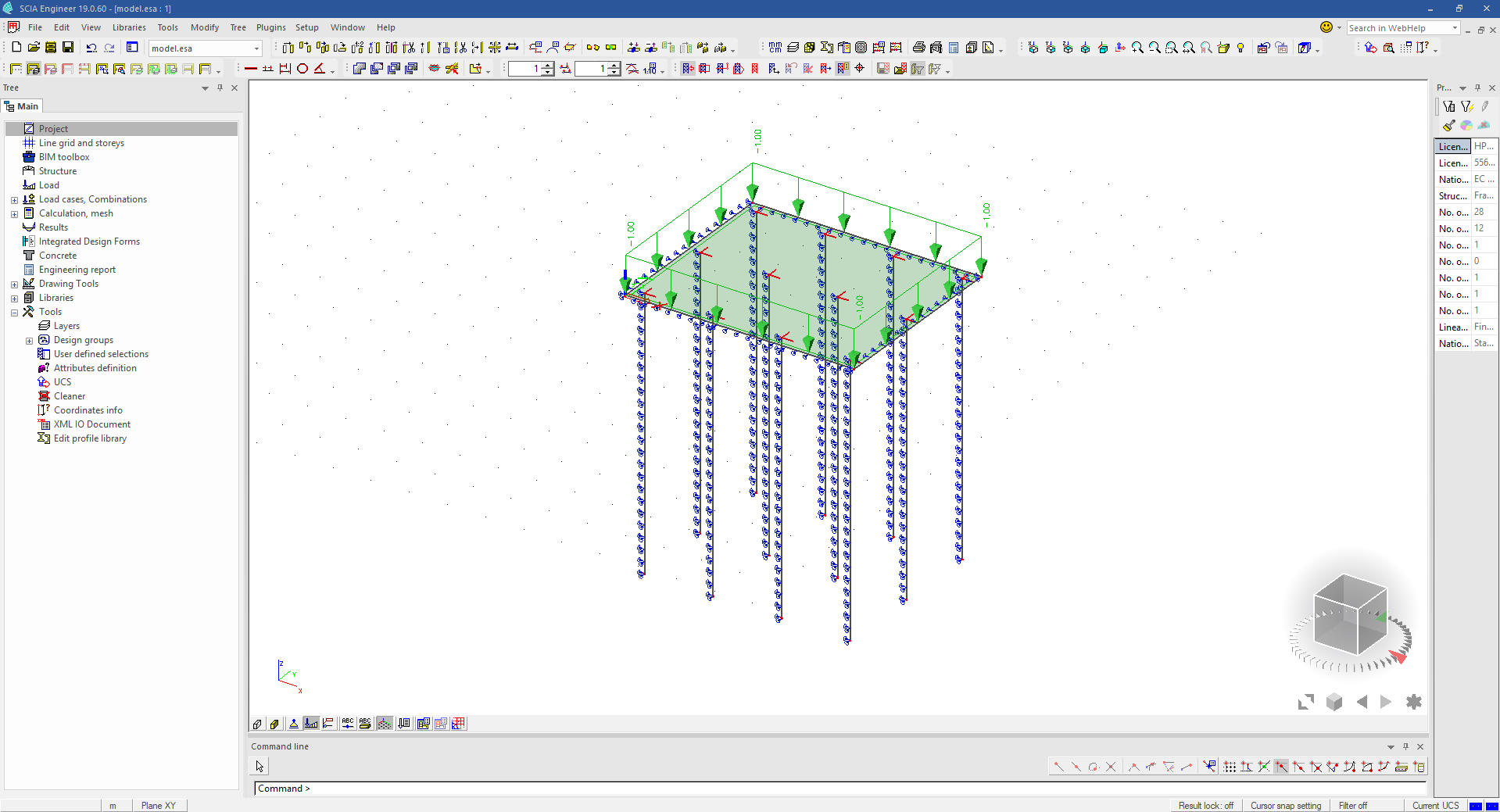SCIA model generated by VIKTOR

## Performing an analysis​

caution

This step requires a SCIA worker.

The three files that are used for the verification above, can now be used as input for the external analysis.

note

An I/O document has to be defined in the `.esa` file, which has to be named "output". If not defined, the worker will not be able to write this expected document and fails to execute.

In this example, the empty `model.esa` has the following structure of the I/O document: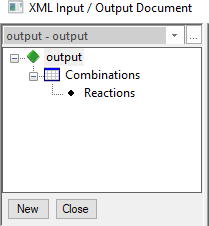I/O document of the esa model

Let's create a second view, which is a combination of geometry and data (results). Set the duration of this view to 60 seconds, which indicates that it concerns a long-running job with a manual update button. This button will be connected to the SCIA analysis:

``...from viktor.external.scia import SciaAnalysisfrom viktor.views import DataGroup, GeometryAndDataView, GeometryAndDataResultclass Foundation(ViktorController):    ...    @GeometryAndDataView("SCIA result", duration_guess=60)    def run_scia(self, params, **kwargs):        scia_model = self.create_scia_model(params)        # create input files        input_xml, input_def = scia_model.generate_xml_input()        input_esa = self.get_scia_input_esa()        # analyze SCIA model        scia_analysis = SciaAnalysis(input_xml, input_def, input_esa)        scia_analysis.execute(300)  # timeout after 5 minutes        data_result = DataGroup()        geometries = self.create_visualization_geometries(params, scia_model)        return GeometryAndDataResult(geometries, data_result)``

After execution, the result can easily be called and parsed as desired.

The "Reactions" results (defined in the I/O document) of the load combination C1 can be parsed from the analysis result using the provided output parser as is shown in the snippet below:

``...from viktor.external.scia import OutputFileParserfrom viktor.views import DataItemclass Foundation(ViktorController):    ...    @GeometryAndDataView(...)    def run_scia(self, params, **kwargs):        scia_model = self.create_scia_model(params)        # create input files        input_xml, input_def = scia_model.generate_xml_input()        input_esa = self.get_scia_input_esa()        # analyze SCIA model        scia_analysis = SciaAnalysis(input_xml, input_def, input_esa)        scia_analysis.execute(300)  # timeout after 5 minutes        scia_result = scia_analysis.get_xml_output_file()        # parse analysis result        result = OutputFileParser.get_result(scia_result, "Reactions", parent='Combinations - C1')        reactions = result['Nodal reactions']        max_rz = float(max(reactions['R_z']))        data_result = DataGroup(            DataItem('SCIA results', ' ', subgroup=DataGroup(                DataItem('Maximum pile reaction', max_rz, suffix='N', number_of_decimals=2)            ))        )        geometries = self.create_visualization_geometries(params, scia_model)        return GeometryAndDataResult(geometries, data_result)``

The pile reactions as seen from within the SCIA interface show a maximum of 3.3 kN (depending on your load input):SCIA analysis result in SCIA

From the result obtained in VIKTOR we can validate that we indeed obtained the maximum reaction force of this load combination: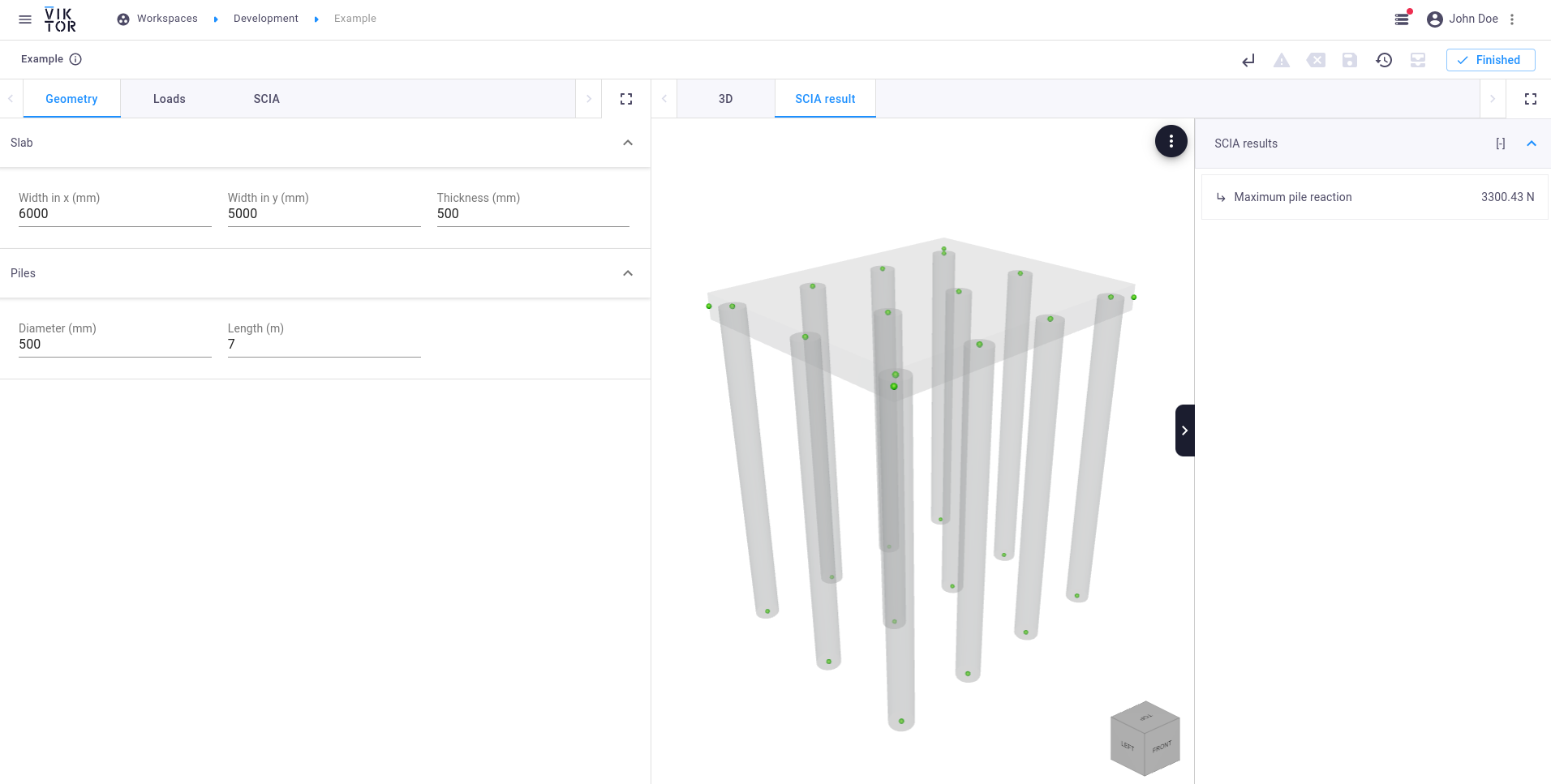SCIA analysis result obtained in VIKTOR